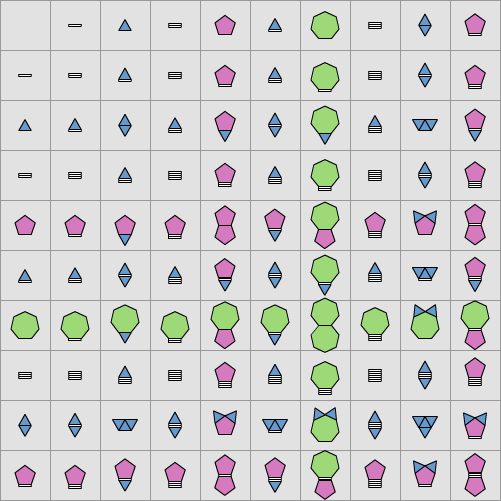# Maths and Physics > Primes-Tables

Don't you wish multiplication was as easy as addition? Well, if you think about it, you'll see that actually it is. The only problem is our need to interpret the result in terms of the number system we're all familiar with. But hey, let's forget that. The idea is simple: every number has a unique prime factorization, e.g. 6 = 2 x 3 and 20 = 2 x 2 x 5. When you multiply two numbers you're just smushing together all the prime factors into one, e.g. 6 x 20 = 2 x 2 x 2 x 3 x 5. Multiplication is just addition of the powers in the prime factorization.

Well that's all fine, so let's make it a bit prettier. Let's draw the prime numbers as shapes we can stick together. We'll choose polygons with number of sides equal to the prime number. So 2 is a line, 3 a triangle, 5 a pentagon, 7 a heptagon etc. Then when we multiply two numbers together we just stick all the shapes together into one. Simple.

Here's what your familiar times tables look like all the way up to 10 x 10:Pretty, but pointless. So, good luck making use of this... especially if you want to do addition! Apologies if you were expecting something profound.